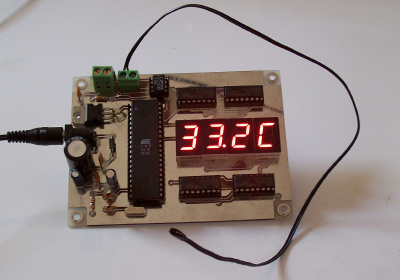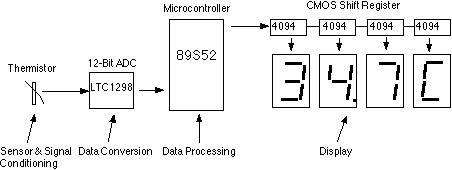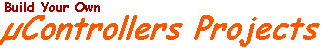Thermistor Thermometer

Wichit Sirichote, wichit.sirichote@gmail.com

Complete student project from sensor to display, built with thermistor, 12-bit ADC, 89S52 and LED display.

This is my student assignment for the class "Designing Microprocessor Based Instrumentation". The board demonstrates the use of 12-bit ADC, writing c program with digital filtering and interface the LED display. The reading provides 0.1C sensitivity. The optional input of the ADC is available for exercise with other input signals or sensors.Figure 1: The prototype of Thermistor Thermometer.

The hardware block diagram is shown in Fig. 2. The sensor is epoxy molded thermistor. The circuit for signal conditioning is simple voltage divider. The ADC is 12-bit SPI interface LTC1298 analog-to-digital converter. The microcontroller is ATMEL 89S52. The display has four digits 0.5 inches 7-segment LED. The segment driver provides 32-bit CMOS output.Figure 2: Hardware block diagram.

Hardware Schematic

The complete hardware schematic is shown in Fig 3. The ADC is 12-bit SPI interface LTC1298 or MC3202. There are two channels, CH0 and CH1. The input signal from thermistor for ADC channel 0 is simple voltage divider. Channel1 is available for other sensor. The sample shown in schematic is HIH-3160 Honeywell Relative Humidity Sensor. The ADC chip is interfaced with MCU, 89S52 with P1.1, P1.2 and P1.3. The display has 4-digit LED. The 4094 CMOS shift register drives the LED directly.

Figure 3: Hardware schematic.

Software

The main function is time triggered by 10ms timer0 running. The ADC is updated on LED every 10 ticks.

 while(1) { while(!cputick) continue; cputick=0; print_ADC(); }

The function that reads 12-bit data from ADC is read_ADC(char n). The function has two loops. First loop is to send 4-bit command. And the second loop is 12-bit to shift the data from ADC.

 ```sbit Data = P1^1;sbit CLK = P1^2;sbit CS = P1^3; int read_ADC(char n) { int k; char i,channel; k=0; CS=0; if(n==0) channel=0x0d; else channel=0x0f;``` ``` for(i=0;i<4;i++) { CLK = 0; if(channel&8) Data = 1; else Data = 0; CLK = 1; channel <<=1; } Data = 1; CLK = 0;``` ``` for(i=0;i<12;i++) { k<<=1; CLK=1; CLK=0; if(Data) k|=1; else k&=~1; } CS = 1; return k&=0xfff; } ```

To provide smooth reading, I added the 5-point moving average to the raw data. The function low_pass_filte1( ) is used to filter the high frequency noise. The reading is calibrated to degree Celsius with Platinum 100 standard thermometer. We found the equation y=0.0323x-15.122.

 `int low_pass_filter1(void){ x5=x4; x4=x3; x3=x2; x2=x1; x1=read_ADC(0); return(x1+x2+x3+x4+x5)/5;}` ```float read_temp1_filter(void) { return(0.0323*low_pass_filter1()-15.122); }```